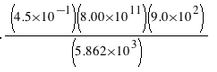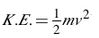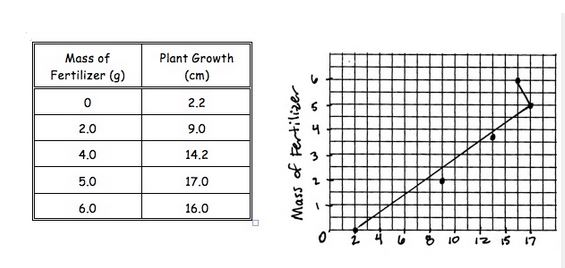# Intro to Physics Review

Highlight areas between ( ) to reveal the contents/answers.

1. Mechanics is the study of (motion). The quantities and units of measure in the MKS system are:

(Highlight the blank squares of the table to reveal the answers)

Quantities Units
distance meter (m)
mass kilogram (kg)
time seconds (s)

2. The units in #1 are called (fundamental) units. They can be combined through calculations into (derived) units. The unit for velocity in the MKS system is the m/s. It is an example of a (derived) unit.

3. What is the difference between weight and mass?
(Mass is constant everywhere. Weight (a special kind of force) changes with location (ex. Earth vs moon).)

4. What belongs in the blank areas of the table? Highlight those areas to reveal the answer.

Decimal Notation Scientific Notation Number of
Significant Digits
3600 3.6 x 103 2
0.000210 2.10 x 10-4 3
0.0002040 2.040 x 10-4 4
60.03 6.003 x 101 4
7,000,000 7.0 x 106 2

5.  Round the following to 3 significant digits:

 4,565,001 4,570,000 0.002101 0.00210

6. Calculate and round to the correct number of digits:8805.5×1010

7. What is the difference between interpolation and extrapolation?
(Interpolation is reading data points within the plotted points, extrapolation is extending the line or curve to read data beyond plotted points.)

8. How do the graphs of direct and inverse proportions differ?
(direct = straight line; inverse = hyperbola)

9. How is a proportion changed into a mathematical equation?
(inserting a constant (and = sign))

10. A formula used often in physics isIf kinetic energy (K.E.) is held constant, mass (m) is (inversely) proportional to velocity squared. IF velocity is held constant, kinetic energy is (directly) proportional to mass.

11. A scientist fed identical plants with different masses of fertilizer and measured the growth of the plants each week. From the data, he constructed a graph. List 5 errors in the graph.(Highlight to reveal possible answers: Variables are on the wrong axis, no labels on x-axis, no units on either axis, no title, not a “best-fit” line, spacing on the x-axis is not consistent)

(source)